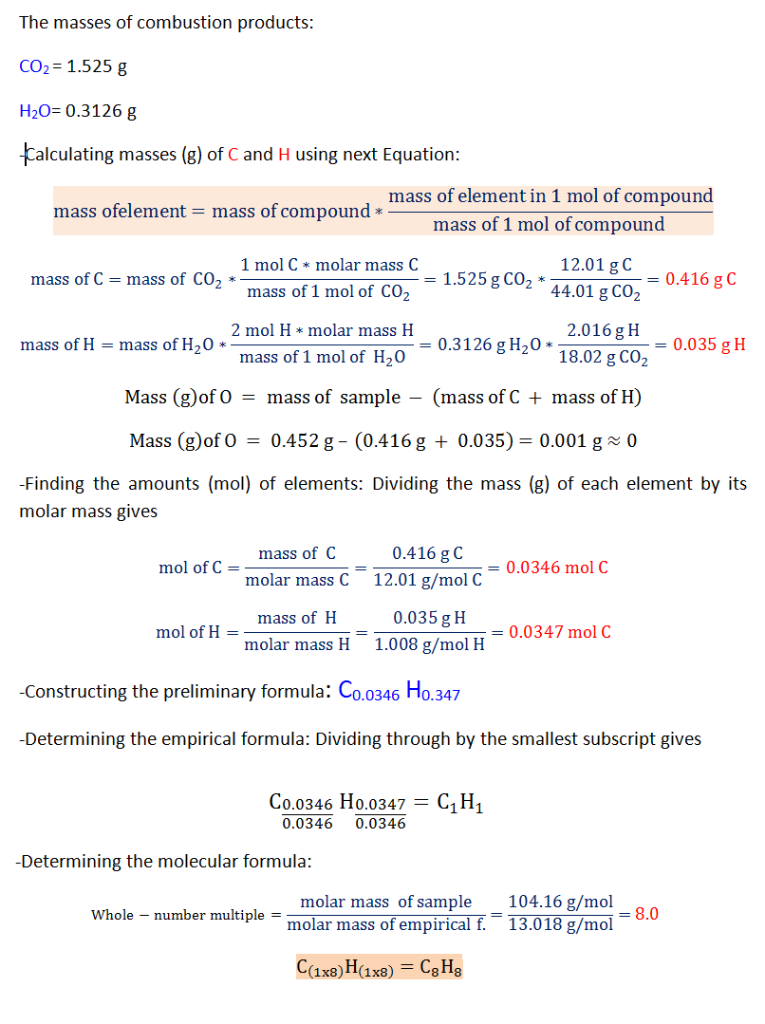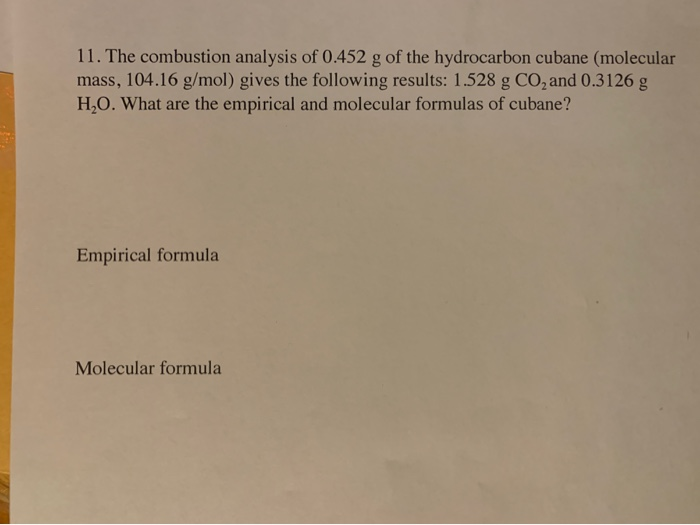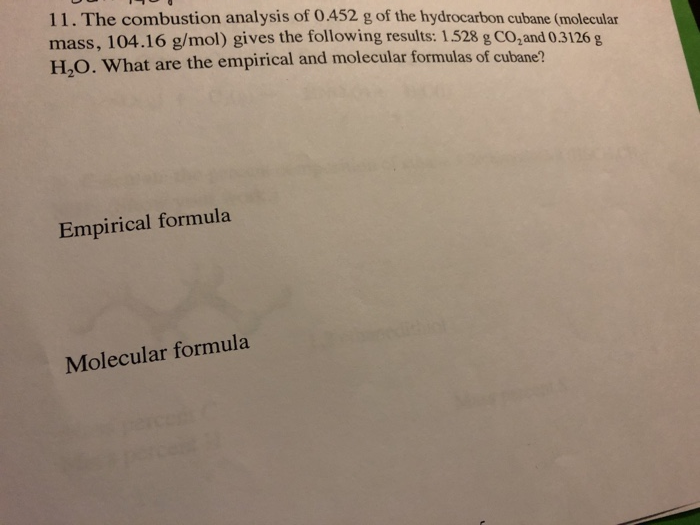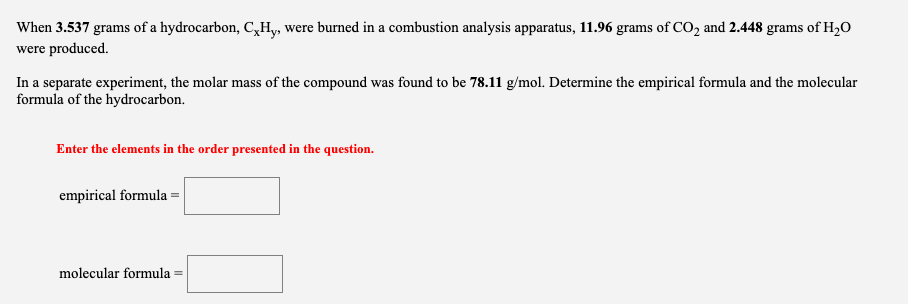Question

# The combustion analysis of 0.452 g of the hydrocarbon cubane (molecular mass, 104.16 g/mol) gives the...

The combustion analysis of 0.452 g of the hydrocarbon cubane (molecular mass, 104.16 g/mol) gives the following results: 1.525 g CO2 and 0.3126 g H2O

What is the empirical and molecular formula of this compound?#### Earn Coins

Coins can be redeemed for fabulous gifts.

Similar Homework Help Questions
• ### 11. The combustion analysis of 0.452 g of the hydrocarbon cubane (molecular mass, 104.16 g/mol) gives...11. The combustion analysis of 0.452 g of the hydrocarbon cubane (molecular mass, 104.16 g/mol) gives the following results: 1.528 g CO, and 0.3126 g H2O. What are the empirical and molecular formulas of cubane? Empirical formula Molecular formula

• ### 11. The combustion analysis of 0.452 g of the hydrocarbon cubane (molecular mass, 104.16 g/mol) gives...11. The combustion analysis of 0.452 g of the hydrocarbon cubane (molecular mass, 104.16 g/mol) gives the following results: 1.528 g CO and 0.3126 g H.O. What are the empirical and molecular formulas of cubane? Empirical formula Molecular formula

• ### A. When 1.591 grams of a hydrocarbon, CxHy, were burned in a combustion analysis apparatus, 5.378...

A. When 1.591 grams of a hydrocarbon, CxHy, were burned in a combustion analysis apparatus, 5.378 grams of CO2 and 1.101 grams of H2O were produced. In a separate experiment, the molar mass of the compound was found to be 26.04 g/mol. Determine the empirical formula and the molecular formula of the hydrocarbon. B. A 9.448 gram sample of an organic compound containing C, H and O is analyzed by combustion analysis and 18.88 grams of CO2 and 7.729 grams...

• ### 1. When 2.689 grams of a hydrocarbon, CxHy, were burned in a combustion analysis apparatus, 8.437...

1. When 2.689 grams of a hydrocarbon, CxHy, were burned in a combustion analysis apparatus, 8.437 grams of CO2 and 3.454 grams of H2O were produced. In a separate experiment, the molar mass of the compound was found to be 56.11 g/mol. Determine the empirical formula and the molecular formula of the hydrocarbon. 2. A 21.79 gram sample of an organic compound containing C, H and O is analyzed by combustion analysis and 21.31 grams of CO2 and 4.362 grams...

• ### When 3.061 grams of a hydrocarbon, CxHy, were burned in a combustion analysis apparatus, 9.603grams of...

When 3.061 grams of a hydrocarbon, CxHy, were burned in a combustion analysis apparatus, 9.603grams of CO2 and 3.932grams of H2O were produced. In a separate experiment, the molar mass of the compound was found to be 42.08 g/mol. Determine the empirical formula and the molecular formula of the hydrocarbon. What is the empirical formula? What is the molecular formula?

• ### 35a When 4.752 grams of a hydrocarbon, CxHy, were burned in a combustion analysis apparatus, 16.06...

35a When 4.752 grams of a hydrocarbon, CxHy, were burned in a combustion analysis apparatus, 16.06 grams of CO2 and 3.289 grams of H2O were produced. In a separate experiment, the molar mass of the compound was found to be 26.04 g/mol. Determine the empirical formula and the molecular formula of the hydrocarbon. 35 part b A 4.079 gram sample of an organic compound containing C, H and O is analyzed by combustion analysis and 9.273 grams of CO2 and...

• ### When 1.696 grams of a hydrocarbon, CxHy, were burned in a combustion analysis apparatus, 5.198 grams...

When 1.696 grams of a hydrocarbon, CxHy, were burned in a combustion analysis apparatus, 5.198 grams of CO2 and 2.483 grams of H2O were produced. In a separate experiment, the molar mass of the compound was found to be 86.18 g/mol. Determine the empirical formula and the molecular formula of the hydrocarbon. Enter the elements in the order presented in the question. empirical formula = molecular formula =

• ### When 1.860 grams of a hydrocarbon, CxHy, were burned in a combustion analysis apparatus, 5.699 grams...

When 1.860 grams of a hydrocarbon, CxHy, were burned in a combustion analysis apparatus, 5.699 grams of CO2 and 2.723 grams of H2O were produced. In a separate experiment, the molar mass of the compound was found to be 86.18 g/mol. Determine the empirical formula and the molecular formula of the hydrocarbon. Enter the elements in the order presented in the question. empirical formula = molecular formula =

• ### When 3.537 grams of a hydrocarbon, C,Hy, were burned in a combustion analysis apparatus, 11.96 grams...When 3.537 grams of a hydrocarbon, C,Hy, were burned in a combustion analysis apparatus, 11.96 grams of CO2 and 2.448 grams of H2O were produced In a separate experiment, the molar mass of the compound was found to be 78.11 g/mol. Determine the empirical formula and the molecular formula of the hydrocarbon. Enter the elements in the order presented in the question. empirical formula = molecular formula =

• ### When 3.741 grams of a hydrocarbon, CxHy, were burned in a combustion analysis apparatus, 12.65 grams...

When 3.741 grams of a hydrocarbon, CxHy, were burned in a combustion analysis apparatus, 12.65 grams of CO2 and 2.589 grams of H2O were produced. In a separate experiment, the molar mass of the compound was found to be 78.11g/mol. Determine the empirical formula and the molecular formula of the hydrocarbon. Enter the elements in the order presented in the question. empirical formula = molecular formula =